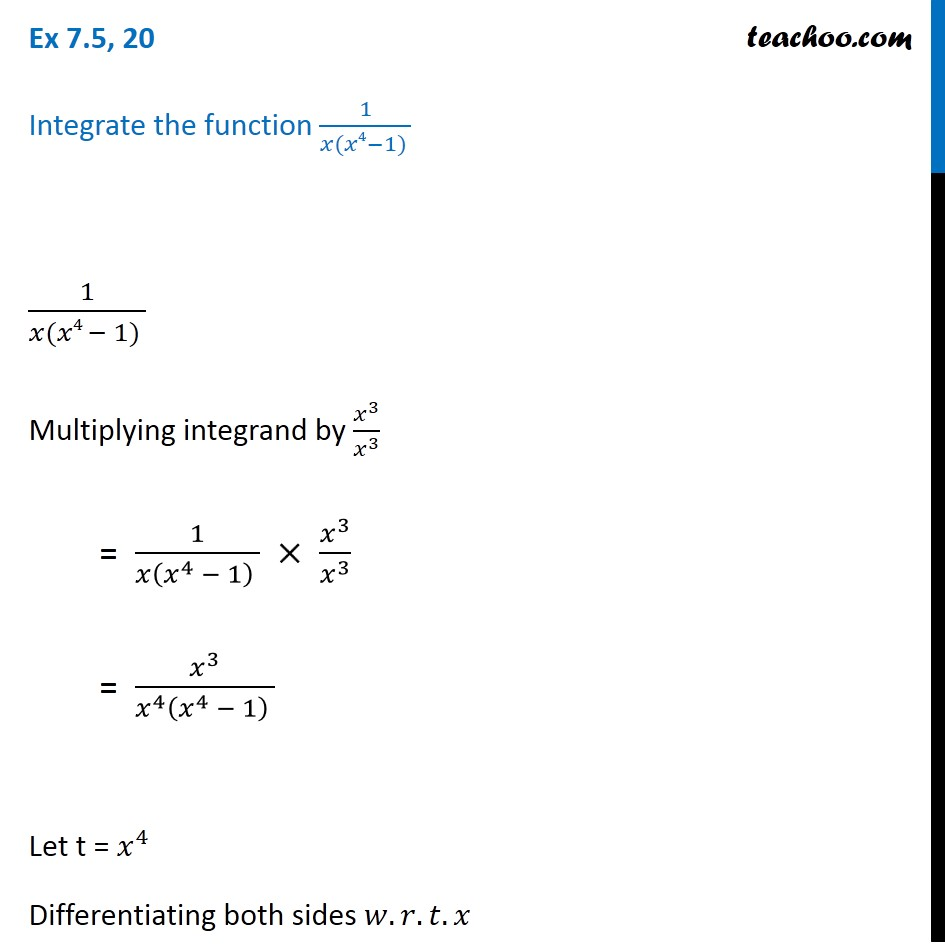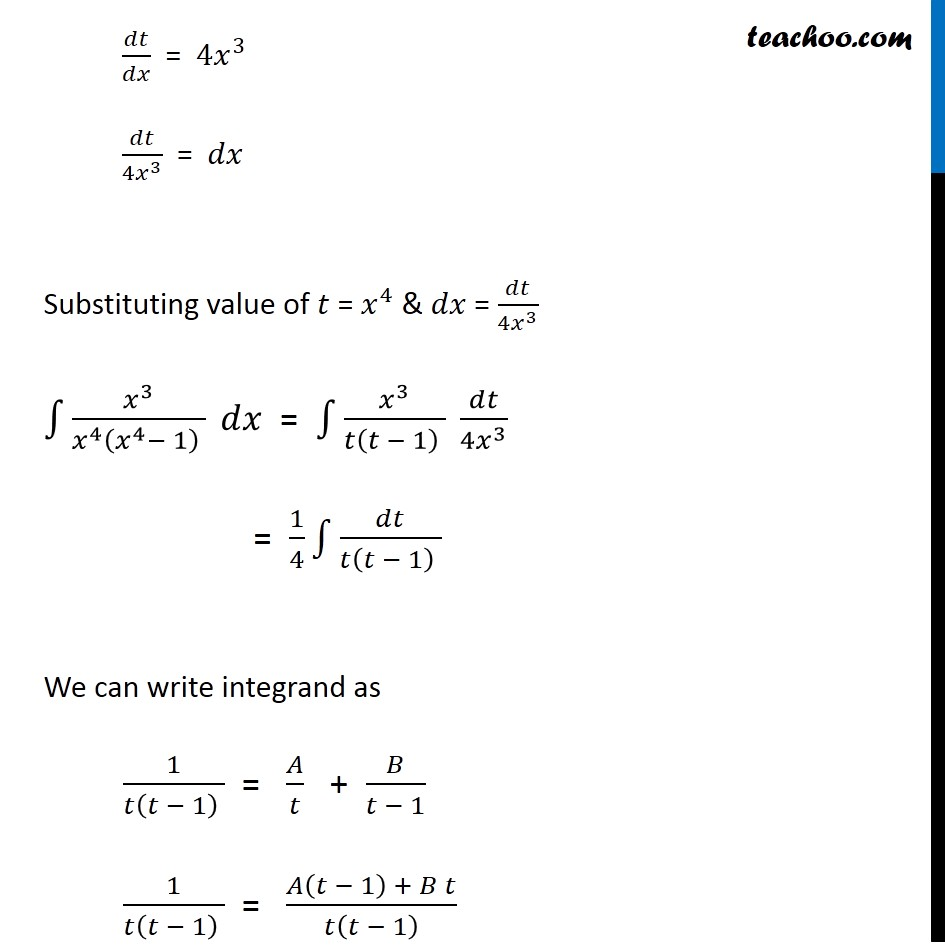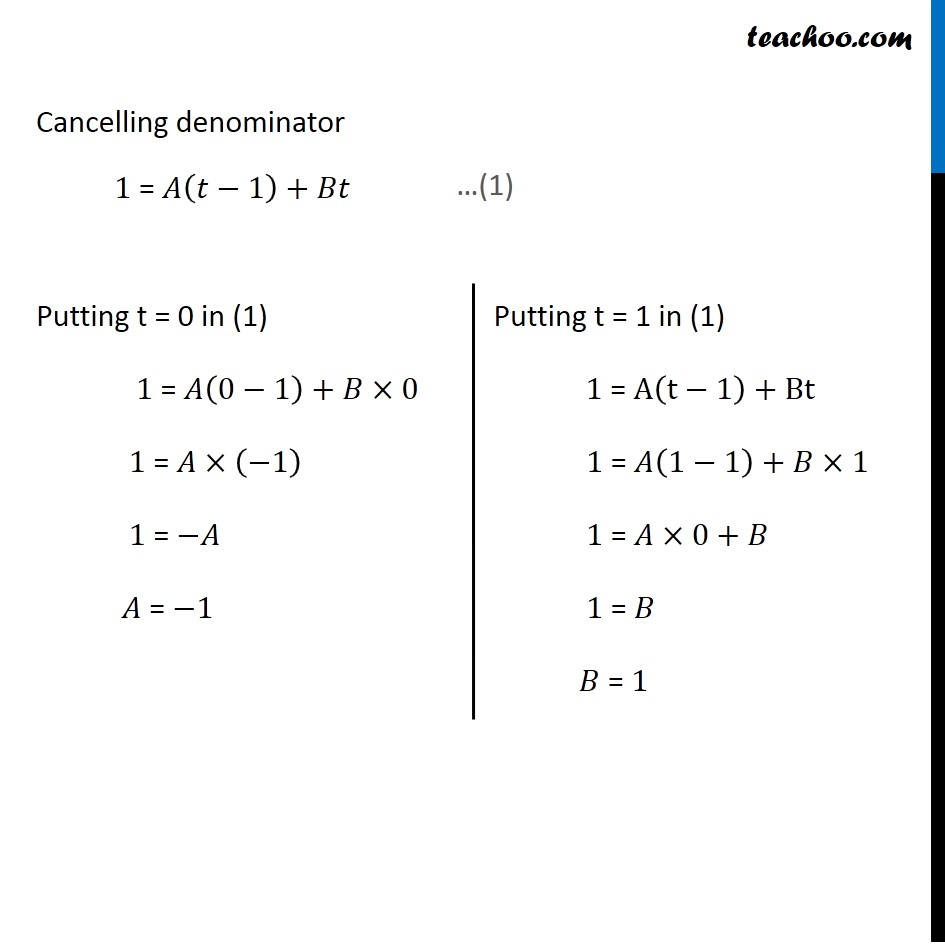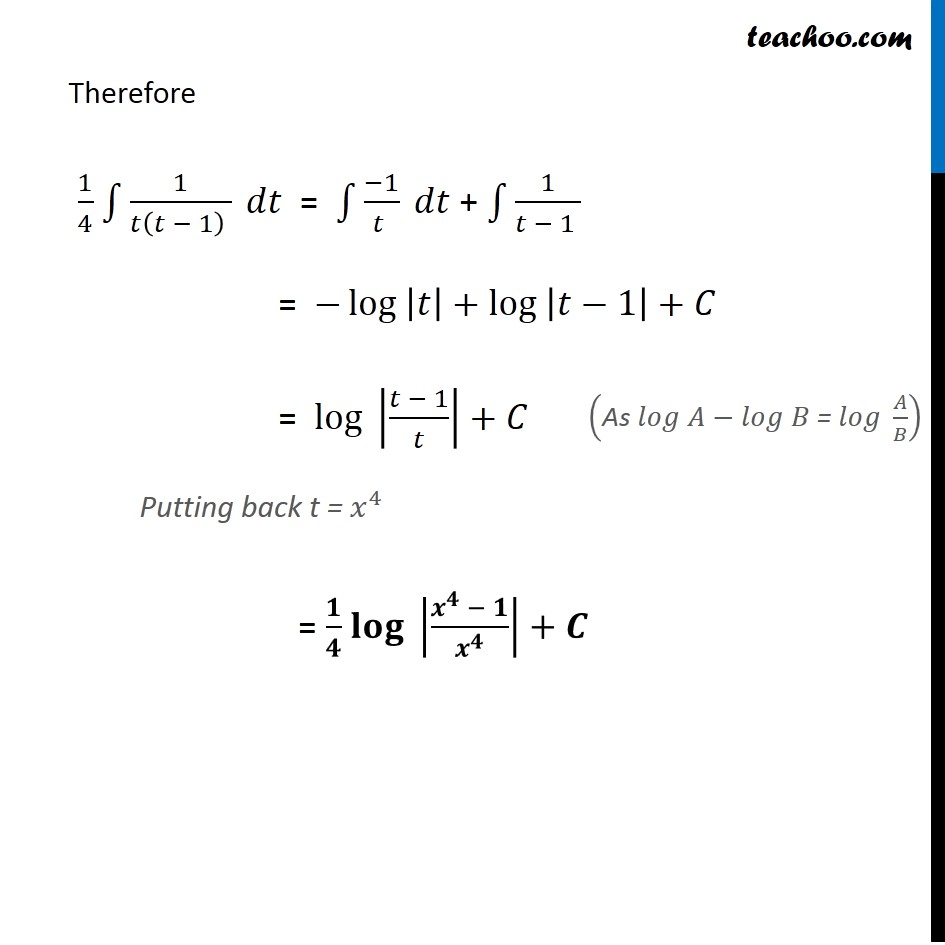Integration by partial fraction - Type 1

Chapter 7 Class 12 Integrals
Concept wiseIntroducing your new favourite teacher - Teachoo Black, at only ₹83 per month

### Transcript

Ex 7.5, 20 Integrate the function 1/(𝑥(𝑥4−1) ) 1/(𝑥(𝑥4 − 1) ) Multiplying integrand by 𝑥^3/𝑥^3 = 1/(𝑥(𝑥^4 − 1) ) × 𝑥^3/𝑥^3 = 𝑥^3/(𝑥^4 (𝑥^4 − 1) ) Let t = 𝑥^4 Differentiating both sides 𝑤.𝑟.𝑡.𝑥 𝑑𝑡/𝑑𝑥 = 4𝑥^3 𝑑𝑡/(4𝑥^3 ) = 𝑑𝑥 Substituting value of 𝑡 = 𝑥^4 & 𝑑𝑥 = 𝑑𝑡/(4𝑥^3 ) " " ∫1▒𝑥^3/(𝑥^4 (𝑥^4− 1) ) 𝑑𝑥 = ∫1▒𝑥^3/(𝑡(𝑡 − 1) ) 𝑑𝑡/(4𝑥^3 ) " " = 1/4 ∫1▒𝑑𝑡/(𝑡(𝑡 − 1) ) We can write integrand as 1/(𝑡(𝑡 − 1) ) = 𝐴/𝑡 + 𝐵/(𝑡 − 1) 1/(𝑡(𝑡 − 1) ) = (𝐴(𝑡 − 1) + 𝐵 𝑡)/𝑡(𝑡 − 1) Cancelling denominator 1 = 𝐴(𝑡−1)+𝐵𝑡 …(1) Putting t = 0 in (1) 1 = 𝐴(0−1)+𝐵×0 1 = 𝐴×(−1) 1 = −𝐴 𝐴 = −1 Putting t = 1 in (1) 1 = A(t−1)+Bt 1 = 𝐴(1−1)+𝐵×1 1 = 𝐴×0+𝐵 1 = 𝐵 𝐵 = 1 Therefore 1/4 ∫1▒1/(𝑡(𝑡 − 1) ) 𝑑𝑡 = ∫1▒(−1)/(𝑡 ) 𝑑𝑡 + ∫1▒1/(𝑡 − 1 ) = −〖log 〗⁡|𝑡|+〖log 〗⁡|𝑡−1|+𝐶 = 〖log 〗⁡|(𝑡 − 1)/𝑡|+𝐶 Putting back t =〖 𝑥〗^4 = 𝟏/𝟒 〖𝐥𝐨𝐠 〗⁡|(𝒙^𝟒 − 𝟏)/𝒙^𝟒 |+𝑪 ("As " 𝑙𝑜𝑔 𝐴−𝑙𝑜𝑔 𝐵" = " 𝑙𝑜𝑔 𝐴/𝐵)